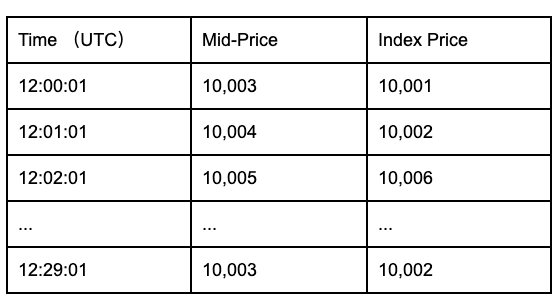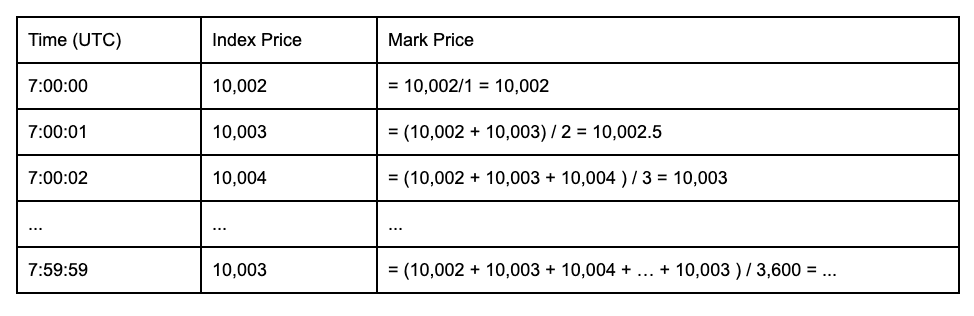English/USD
Language
Currency
Support Center
FAQ
Crypto Derivatives
Futures Contracts
Quarterly Futures Contract
Mark Price in Quarterly Futures Contract
Binance
2020-06-11 07:38

Important Note: Binance Futures uses a mark price as a reference in liquidations and calculations of unrealized PNL. Mark price is an estimated fair value of a contract and it differs from ‘last price’. A mark price is used to prevent unfair and unnecessary liquidations that may happen when the market is highly volatile. Additionally, it also helps to prevent price manipulation.

It is important to note that mark prices in both perpetual futures and quarterly futures are different and they are computed with different formulas and methodologies. We highly recommended reading both sections (Mark Price in Perpetual Futures and Mark Price in Quarterly Futures) to get a clear understanding of mark price methodologies.

A Mark Price consists of two components: Index Price and Moving Average(MA) Basis.

Index Price is an aggregate price extracted from the major spot exchanges, weighted by their relative volume, this is done to prevent price manipulation from a single exchange. The Index Price for BTCUSD Quarterly 0925 contracts derived prices from Bitstamp, Coinbase Pro(GDAX), Kraken, Bittrex, and Binance.

A moving average basis is used as the second component of mark price calculation. It helps to smooth out the price data over a specified period of time by creating a constantly updated average price. This methodology reduces the possibility of unfair and unnecessary liquidations when the market is highly volatile.

Traditionally, the price of a quarterly futures contract will converge with its corresponding spot price as the contract expires after the three-month period. As the contract runs down towards expiry, the mark price will closely reflect spot prices and the moving average basis component will no longer be part of the mark price calculation. This means that the mark price of a quarterly futures contract will be computed differently as it reaches the time of expiration.

On the expiration date, the mark price for a quarterly futures contract will be calculated as follows:

i) The time to delivery is greater than 1 hour

Formula

Mark Price before 25 September 2020, 06:59:59 UTC

=  Index Price + Moving Average (30-minute Basis)*

*Moving Average (30-minute Basis) = Moving Average ((Bid1+Ask1)/2- Index Price), which measures every minute in 30-minute interval

How to Calculate

Step 1: Calculate the Index Price

Assume Binance uses an equally-weighted price average, the prices of BTCUSD trading pairs on the selected exchanges are 10,000 USD, 10,0001 USD, 10,0002 USD, 10,003 USD and 10,004 USD respectively.

Index Price = (10,000 + 10,001 + 10,002 + 10,003 + 10,004) / 5 = 10,002 USD

Step 2: Calculate the Moving Average on 30-minute Basis

Moving Average (30-minute Basis)

= Moving Average (Mid-Price*- Index Price), which measures every minute in 30-minute interval

To calculate moving average, we need to get the mid-price from the order book and index price of the first second of every minute for the past 30 minutes, then we will have n=30 in total.

For example, if we want to calculate the Mark Price of BTCUSD 0925 at 12.30:00 UTC, the mid-prices and index prices are as follows:Moving Average (30-minute Basis)

= Moving Average (Mid-Price- Index Price)

= [(Mid-Price- Index Price)1 + (Mid-Price- Index Price)2 + … + (Mid-Price- Index Price)30 ] / 30

= [(10,003 - 10,001) + (10,004 - 10,002) + … + (10,005 - 10,006)] / 30

Step 3: Substitute the Index Price and Moving Average (30-minute Basis) into the formula

Let say Index Price = 10,002 USD and Moving Average (30-minute Basis) = -1

Mark Price at 12.30:00 UTC

=  Index Price + Moving Average (30-minute Basis)

= 10,002 USD - 1 USD

= 10,001 USD

ii) Time to delivery is equal or less than 1 hour

Formula

Mark Price on 25 September 2020, 07:00:00 - 07:59:59 UTC

= Average of Index Price (every second from 07:00:00 and 07:59:59 UTC on the delivery day)

How to Calculate

Step 1: Calculate the Index Price

Assume Binance uses an equally-weighted price average, the prices of BTCUSD trading pairs on the selected exchanges are 10,000 USD, 10,0001 USD, 10,0002 USD, 10,003 USD and 10,004 USD respectively.

Index Price = (10,000 + 10,001 + 10,002 + 10,003 + 10,004) / 5 = 10,002 USD

Step 2: Calculate the Average of Index Price

Mark Price at Timen

= (Index Price1 + Index Price2 + … + Index Pricen) / n

Example:

Mark Price at 7:00:02 on 25 September

= (Index Price at 7:00:00 + Index Price at 7:00:01 + Index Price at 7:00:02) / 3

= (10,002 + 10,003 + 10,004) / 3

= 10,003Have more questions?submit a request
Related Articles